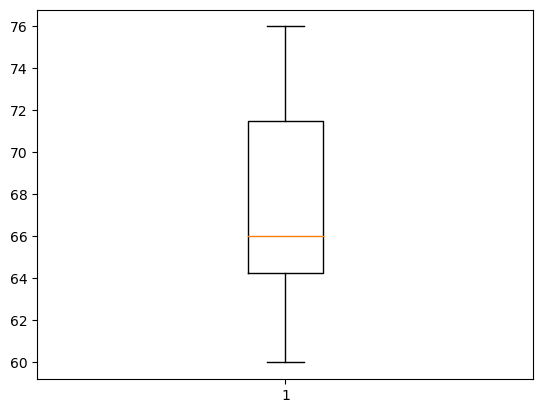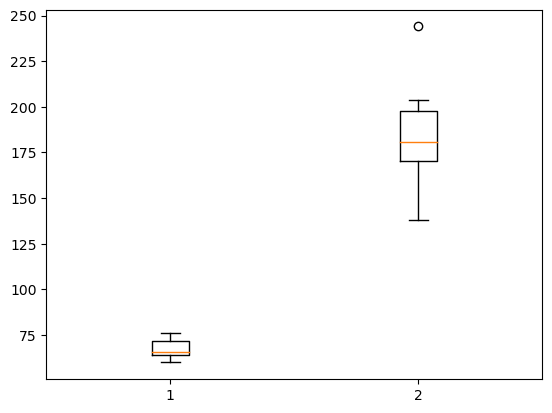# How to create a box (and whisker) plot

## Description

A box plot, or a box and whisker plot, shows the quartiles of a single variable from a dataset (one of which is the median) and may also show the outliers. It is a simplified way to see the distribution of a variable. Sometimes multiple box plots (one for each of several variables) are shown side-by-side on a plot, to compare the variables. How can we create such graphs?

Related topics:

## Using Matplotlib, in Python

View this solution alone.

We will create some fake data using Python lists, for simplicity. But everything we show below works also if your data is in columns of a DataFrame, such as df['age'].

1
2
3
patient_id     = [   0,   1,   2,   3,   4,   5,   6,   7,   8,   9 ]
patient_height = [  60,  64,  64,  65,  66,  66,  70,  72,  72,  76 ]
patient_weight = [ 141, 182, 169, 204, 138, 198, 180, 175, 244, 196 ]


The conventional way to import matplotlib in Python is as follows.

1
import matplotlib.pyplot as plt


To create a box-and-whisker plot, sometimes called just a box plot requires just one line of code, plus one to show the plot.

1
2
plt.boxplot( patient_height )
plt.show()You can show more than one variable’s box plot side-by-side by forming a list of the data.

1
2
plt.boxplot( [ patient_height, patient_weight ] )
plt.show()See a problem? Tell us or edit the source.

## Solution, in R

View this solution alone.

We will create some fake data using vectors, for simplicity. But everything we show below works also if your data is in columns of a DataFrame.

1
2
3
patient_id     <- c(0,   1,   2,   3,   4,   5,   6,   7,   8,   9)
patient_height <- c(60,  64,  64,  65,  66,  66,  70,  72,  72,  76)
patient_weight <- c(141, 182, 169, 204, 138, 198, 180, 175, 244, 196)


We can use R’s boxplot() function to make the plot.

1
boxplot(patient_weight)


You can show more than one variable’s box plot side-by-side by passing both variables into the boxplot() function.

1
boxplot(patient_height, patient_weight)